## Example Questions

← Previous 1 3 4 5 6 7 8 9 38 39

### Example Question #1 : Plane GeometryIn the figure above, lines AB and CD are parallel. Also, a, b, c, d, e, f, g, and h represent the measures of the angles in which they are shown. All of the following must be true EXCEPT:

f + d = a + g

b – h = e – c

180 – a – c = e + g – 180

a + h = e + d

f = b

b – h = e – c

Explanation:

Lines AB and CD are parallel, so that means that d and e, which are in alternate interior angles, must be equal. Because a and d are vertical angles, and because e and h are vertical angles, this means that a = d and e = h. Therefore, a = d = e = h. Similarly, b = c = f = g.

Let us look at the choice f = b. We can see that this is true because b, c, f, and g are all equal.

We can then look at f + d = a + g. Let us subtract g from both sides, and then let us subtract d from both sides. This would give us the following equation.

f – g = a – d

Because f and g are equal, f – g = 0. Also, because a and d are the same, a – d = 0. Therefore, f – g = a – d = 0, so this is always true.

Now, let's look at 180 – a – c = e + g - 180. We can rewrite this as:

180 – (a + c) = e + g – 180.

a and c are supplementary, so a + c = 180. Likewise, e + g = 180. We can substitute 180 in for a + c and for e + g.

180 – 180 = 180 – 180 = 0

This means that 180 – a – c = e + g – 180 is indeed always true.

Next, let's examine the choice a + h = e + d. Let us subtract e from both sides and h from both sides. This will give us the following:

a – e = d – h

Because a = e = d = h, we could replace all of the values with a.

a – a = a – a = 0, so this is always true.

The final choice is b – h = e – c. Let us substitute c in for b and h in for e.

c – h = h – c

Let us add c and h to both sides.

2c = 2h

This means that c must equal h for this to be true. However, c does not always have to equal h. We know that c must equal f, and we know that f + h must equal 180. This means that c + h must equal 180. But this doesn't necessarily mean that c must equal h. In fact, this will only be true if c and h are both 90. Therefore, b – h = e – c isn't always true.

The answer is b – h = e – c.

### Example Question #1 : Lines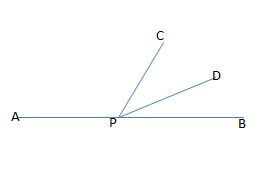Figure not drawn to scale.

In the figure above, APB forms a straight line. If the measure of angle APC is eighty-one degrees larger than the measure of angle DPB, and the measures of angles CPD and DPB are equal, then what is the measure, in degrees, of angle CPB?

114

40

33

50

66

66

Explanation:

Let x equal the measure of angle DPB. Because the measure of angle APC is eighty-one degrees larger than the measure of DPB, we can represent this angle's measure as x + 81. Also, because the measure of angle CPD is equal to the measure of angle DPB, we can represent the measure of CPD as x.

Since APB is a straight line, the sum of the measures of angles DPB, APC, and CPD must all equal 180; therefore, we can write the following equation to find x:

x + (x + 81) + x = 180

Simplify by collecting the x terms.

3x + 81 = 180

Subtract 81 from both sides.

3x = 99

Divide by 3.

x = 33.

This means that the measures of angles DPB and CPD are both equal to 33 degrees. The original question asks us to find the measure of angle CPB, which is equal to the sum of the measures of angles DPB and CPD.

measure of CPB = 33 + 33 = 66.

### Example Question #1 : Geometry

One-half of the measure of the supplement of angle ABC is equal to the twice the measure of angle ABC. What is the measure, in degrees, of the complement of angle ABC?

36

72

90

54

18

54

Explanation:

Let x equal the measure of angle ABC, let y equal the measure of the supplement of angle ABC, and let z equal the measure of the complement of angle ABC.

Because x and y are supplements, the sum of their measures must equal 180. In other words, x + y = 180.

We are told that one-half of the measure of the supplement is equal to twice the measure of ABC. We could write this equation as follows:

(1/2)y = 2x.

Because x + y = 180, we can solve for y in terms of x by subtracting x from both sides. In other words, y = 180 – x. Next, we can substitute this value into the equation (1/2)y = 2x and then solve for x.

(1/2)(180-x) = 2x.

Multiply both sides by 2 to get rid of the fraction.

(180 – x) = 4x.

180 = 5x.

Divide both sides by 5.

x = 36.

The measure of angle ABC is 36 degrees. However, the original question asks us to find the measure of the complement of ABC, which we denoted previously as z. Because the sum of the measure of an angle and the measure of its complement equals 90, we can write the following equation:

x + z = 90.

Now, we can substitute 36 as the value of x and then solve for z.

36 + z = 90.

Subtract 36 from both sides.

z = 54.

### Example Question #1 : Plane Geometry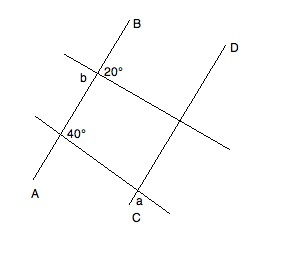In the diagram, AB || CD. What is the value of a+b?

160°

140°

80°

60°

160°

Explanation:

Refer to the following diagram while reading the explanation: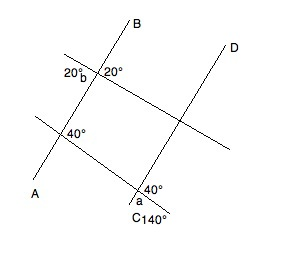We know that angle b has to be equal to its vertical angle (the angle directly "across" the intersection).  Therefore, it is 20°.

Furthermore, given the properties of parallel lines, we know that the supplementary angle to a must be 40°.  Based on the rule for supplements, we know that a + 40° = 180°.  Solving for a, we get a = 140°.

Therefore, a + b = 140° + 20° = 160°

### Example Question #2 : Geometry

In rectangle ABCD, both diagonals are drawn and intersect at point E.

Let the measure of angle AEB equal x degrees.

Let the measure of angle BEC equal y degrees.

Let the measure of angle CED equal z degrees.

Find the measure of angle AED in terms of x, y, and/or z.

180 – y

180 – 2(x + z)

360 – x + y + z

180 – (x + y + z)

180 – 1/2(x + z)

180 – 1/2(x + z)

Explanation:

Intersecting lines create two pairs of vertical angles which are congruent. Therefore, we can deduce that y = measure of angle AED.

Furthermore, intersecting lines create adjacent angles that are supplementary (sum to 180 degrees). Therefore, we can deduce that x + y + z + (measure of angle AED) = 360.

Substituting the first equation into the second equation, we get

x + (measure of angle AED) + z + (measure of angle AED) = 360

2(measure of angle AED) + x + z = 360

2(measure of angle AED) = 360 – (x + z)

Divide by two and get:

measure of angle AED = 180 – 1/2(x + z)

### Example Question #251 : Geometry

A student creates a challenge for his friend.  He first draws a square, the adds the line for each of the 2 diagonals.  Finally, he asks his friend to draw the circle that has the most intersections possible.

How many intersections will this circle have?Explanation:

### Example Question #1 : Plane Geometry

Two pairs of parallel lines intersect: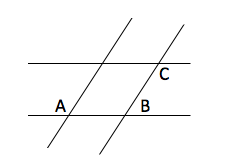If A = 135o, what is 2*|B-C| = ?

170°

140°

150°

160°

180°

180°

Explanation:

By properties of parallel lines A+B = 180o, B = 45o, C = A = 135o, so 2*|B-C| = 2* |45-135| = 180o

### Example Question #1 : Geometry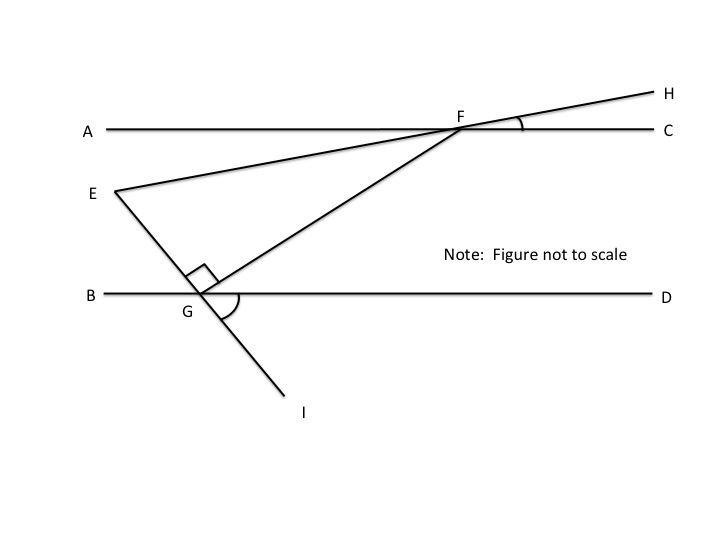Lines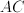and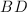are parallel.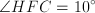,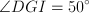,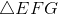is a right triangle, and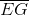has a length of 10.  What is the length of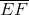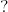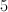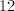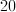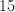Not enough information.Explanation:

Since we know opposite angles are equal, it follows that angle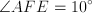and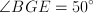.

Imagine a parallel line passing through point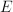.  The imaginary line would make opposite angles with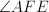&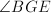, the sum of which would equal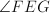.  Therefore,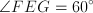.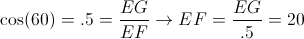### Example Question #1 : Geometry

If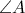measures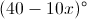, which of the following is equivalent to the measure of the supplement of?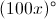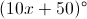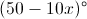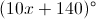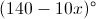Explanation:

When the measure of an angle is added to the measure of its supplement, the result is always 180 degrees. Put differently, two angles are said to be supplementary if the sum of their measures is 180 degrees. For example, two angles whose measures are 50 degrees and 130 degrees are supplementary, because the sum of 50 and 130 degrees is 180 degrees. We can thus write the following equation: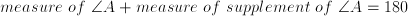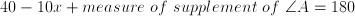Subtract 40 from both sides.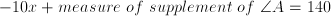Add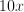to both sides.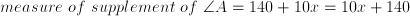The answer is.

### Example Question #1 : How To Find The Angle Of Two Lines

In the following diagram, linesandare parallel to each other. What is the value for?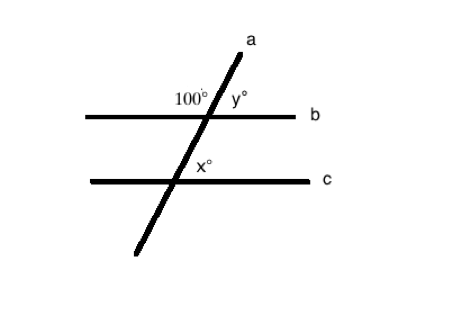It cannot be determined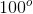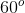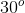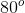Explanation:

When two parallel lines are intersected by another line, the sum of the measures of the interior angles on the same side of the line is 180°. Therefore, the sum of the angle that is labeled as 100° and angle y is 180°. As a result, angle y is 80°.

Another property of two parallel lines that are intersected by a third line is that the corresponding angles are congruent. So, the measurement of angle x is equal to the measurement of angle y, which is 80°.

← Previous 1 3 4 5 6 7 8 9 38 39

### All SAT Math Resources